xSulba SutrasEncyclopedia
The Shulba Sutras or Śulbasūtras (Sanskrit
Sanskrit
Sanskrit , is a historical Indo-Aryan language and the primary liturgical language of Hinduism, Jainism and Buddhism.Buddhism: besides Pali, see Buddhist Hybrid Sanskrit Today, it is listed as one of the 22 scheduled languages of India and is an official language of the state of Uttarakhand...

: "string, cord, rope") are sutra
Sutra
Sūtra is an aphorism or a collection of such aphorisms in the form of a manual. Literally it means a thread or line that holds things together and is derived from the verbal root siv-, meaning to sew , as does the medical term...

texts belonging to the Śrauta
Srauta
' traditions are conservative ritualistic traditions of the historical Vedic religion in Hinduism, based on the body of Śruti literature...

ritual and containing geometry related to fire-altar construction.

## Purpose and origins

The Shulba Sutras are part of the larger corpus of texts called the Shrauta Sutras, considered to be appendices to the Vedas
Vedas
The Vedas are a large body of texts originating in ancient India. Composed in Vedic Sanskrit, the texts constitute the oldest layer of Sanskrit literature and the oldest scriptures of Hinduism....

. They are the only sources of knowledge of Indian mathematics
Indian mathematics
Indian mathematics emerged in the Indian subcontinent from 1200 BCE until the end of the 18th century. In the classical period of Indian mathematics , important contributions were made by scholars like Aryabhata, Brahmagupta, and Bhaskara II. The decimal number system in use today was first...

from the Vedic period
Vedic period
The Vedic period was a period in history during which the Vedas, the oldest scriptures of Hinduism, were composed. The time span of the period is uncertain. Philological and linguistic evidence indicates that the Rigveda, the oldest of the Vedas, was composed roughly between 1700–1100 BCE, also...

. Unique fire-altar shapes were associated with unique gifts from the Gods. For instance, "he who desires heaven is to construct a fire-altar in the form of a falcon"; "a fire-altar in the form of a tortoise is to be constructed by one desiring to win the world of Brahman" and "those who wish to destroy existing and future enemies should construct a fire-altar in the form of a rhombus".

The four major Shulba Sutras, which are mathematically the most significant, are those composed by Baudhayana
Baudhayana
Baudhāyana, was an Indian mathematician, whowas most likely also a priest. He is noted as the author of the earliest Sulba Sūtra—appendices to the Vedas giving rules for the construction of altars—called the , which contained several important mathematical results. He is older than the other...

, Manava
Manava
Manava is an author of the Indian geometric text of Sulba Sutras.The Manava Sulbasutra is not the oldest , nor is it one of the most important, there being at least three Sulbasutras which are considered more important...

, Apastamba
Apastamba
The Dharmasutra of Āpastamba forms a part of the larger Kalpasūtra of Āpastamba. It contains thirty praśnas, which literally means ‘questions’ or books. The subjects of this Dharmasūtra are well organized and preserved in good condition...

and Katyayana, about whom very little is known. The texts are dated by comparing their grammar and vocabulary with the grammar and vocabulary of other Vedic texts. The texts have been dated from around 800 BCE to 200
200
Year 200 was a leap year starting on Tuesday of the Julian calendar. At the time, it was known as the Year of the Consulship of Severus and Victorinus...

CE, with the oldest being the sutra that was written by Baudhayana around 800 BCE to 600 BCE.

There are competing theories about the origin of the geometry that is found in the Shulba sutras, and of geometry in general. According to the theory of the ritual origins of geometry, different shapes symbolized different religious ideas, and the need to manipulate these shapes lead to the creation of the pertinent mathematics. Another theory is that the mystical properties of numbers and geometry were considered spiritually powerful and consequently, led to their incorporation into religious texts.

### Pythagorean theorem

The sutras contain discussion and non-axiomatic demonstrations of cases of the Pythagorean theorem
Pythagorean theorem
In mathematics, the Pythagorean theorem or Pythagoras' theorem is a relation in Euclidean geometry among the three sides of a right triangle...

and Pythagorean triples. It is also implied and cases presented in the earlier work of Apastamba
Apastamba
The Dharmasutra of Āpastamba forms a part of the larger Kalpasūtra of Āpastamba. It contains thirty praśnas, which literally means ‘questions’ or books. The subjects of this Dharmasūtra are well organized and preserved in good condition...

and Baudhayana
Baudhayana
Baudhāyana, was an Indian mathematician, whowas most likely also a priest. He is noted as the author of the earliest Sulba Sūtra—appendices to the Vedas giving rules for the construction of altars—called the , which contained several important mathematical results. He is older than the other...

, although there is no consensus on whether or not Apastamba's rule is derived from Mesopotamia. In Baudhayana, the rules are given as follows:
1.9. The diagonal of a square produces double the area [of the square].
[...]
1.12. The areas [of the squares] produced separately by the lengths of the breadth of a rectangle together equal the area [of the square] produced by the diagonal.
1.13. This is observed in rectangles having sides 3 and 4, 12 and 5, 15 and 8, 7 and 24, 12 and 35, 15 and 36.

The Satapatha Brahmana and the Taittiriya Samhita were probably also aware of the Pythagoras theorem. Seidenberg (1983) argued that either "Old Babylonia got the theorem of Pythagoras from India or that Old Babylonia and India got it from a third source". Seidenberg suggested that this source might be Sumer
Sumer
Sumer was a civilization and historical region in southern Mesopotamia, modern Iraq during the Chalcolithic and Early Bronze Age....

ian and may predate 1700 BC.

### Pythagorean triples

Pythagorean triples are found in Apastamba
Apastamba
The Dharmasutra of Āpastamba forms a part of the larger Kalpasūtra of Āpastamba. It contains thirty praśnas, which literally means ‘questions’ or books. The subjects of this Dharmasūtra are well organized and preserved in good condition...

's rules for altar construction. They were used for the construction of right angles. The complete list is:
•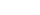•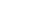•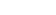•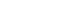However, since these triples are easily derived from an old Babylonian rule, Mesopotamian influence is not unlikely.

### Geometry

The Baudhayana Shulba sutra gives the construction of geometric shapes such as squares and rectangles. It also gives, sometimes approximate, geometric area-preserving transformations from one geometric shape to another. These include transforming a square
Square (geometry)
In geometry, a square is a regular quadrilateral. This means that it has four equal sides and four equal angles...

into a rectangle
Rectangle
In Euclidean plane geometry, a rectangle is any quadrilateral with four right angles. The term "oblong" is occasionally used to refer to a non-square rectangle...

, an isosceles trapezium
Trapezium
The word trapezium has several meanings:* - a quadrilateral with one pair of parallel sides ....

, an isosceles triangle
Triangle
A triangle is one of the basic shapes of geometry: a polygon with three corners or vertices and three sides or edges which are line segments. A triangle with vertices A, B, and C is denoted ....

, a rhombus
Rhombus
In Euclidean geometry, a rhombus or rhomb is a convex quadrilateral whose four sides all have the same length. The rhombus is often called a diamond, after the diamonds suit in playing cards, or a lozenge, though the latter sometimes refers specifically to a rhombus with a 45° angle.Every...

, and a circle
Circle
A circle is a simple shape of Euclidean geometry consisting of those points in a plane that are a given distance from a given point, the centre. The distance between any of the points and the centre is called the radius....

, and transforming a circle into a square. In these texts approximations, such as the transformation of a circle into a square, appear side by side with more accurate statements. As an example, the statement of circling the square is given in Baudhayana as:
2.9. If it is desired to transform a square into a circle, [a cord of length] half the diagonal [of the square] is stretched from the centre to the east [a part of it lying outside the eastern side of the square]; with one-third [of the part lying outside] added to the remainder [of the half diagonal], the [required] circle is drawn.

and the statement of squaring the circle is given as:
2.10. To transform a circle into a square, the diameter is divided into eight parts; one [such] part after being divided into twenty-nine parts is reduced by twenty-eight of them and further by the sixth [of the part left] less the eighth [of the sixth part].
2.11. Alternatively, divide [the diameter] into fifteen parts and reduce it by two of them; this gives the approximate side of the square [desired].

The constructions in 2.9 and 2.10 give a value of π as 3.088, while the construction in 2.11 gives π as 3.004.

### Square roots

Altar construction also led to an estimation of the square root of 2
Square root of 2
The square root of 2, often known as root 2, is the positive algebraic number that, when multiplied by itself, gives the number 2. It is more precisely called the principal square root of 2, to distinguish it from the negative number with the same property.Geometrically the square root of 2 is the...

as found in three of the sutras. In the Baudhayana sutra it appears as:
2.12. The measure is to be increased by its third and this [third] again by its own fourth less the thirty-fourth part [of that fourth]; this is [the value of] the diagonal of a square [whose side is the measure].

which leads to the value of the square root of two as being: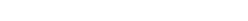One conjecture about how such an approximation was obtained is that it was taken by the formula: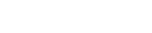with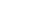and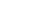which is an approximation that follows a rule given by the twelfth century Muslim mathematician Al-Hassar. The result is correct to 5 decimal places.

This formula is also similar in structure to the formula found on a Mesopotamian tablet from the Old Babylonian period (1900-1600 BCE):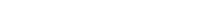which expresses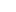in the sexagesimal system, and which too is accurate up to 5 decimal places (after rounding).

Indeed an early method for calculating square roots can be found in some Sutras, the method involves the recursive
Recursion
Recursion is the process of repeating items in a self-similar way. For instance, when the surfaces of two mirrors are exactly parallel with each other the nested images that occur are a form of infinite recursion. The term has a variety of meanings specific to a variety of disciplines ranging from...

formula: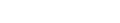for large values of x, which bases itself on the non-recursive identity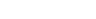for values of r extremely small relative to a.

### Numerals

Before the period of the Sulbasutras was at an end, the Brahmi numeral
Brahmi numeral
The Brahmi numerals are an indigenous Indian numeral system attested from the 3rd century BCE . They are the direct graphic ancestors of the modern Indic and Hindu-Arabic numerals. However, they were conceptually distinct from these later systems, as they were not used as a positional system with a...

s had definitely begun to appear (c. 300BCE) and the similarity with modern day numerals is clear to see. More importantly even still was the development of the concept of decimal place value. Certain rules given by the famous Indian grammarian Pāṇini (c. 500 BCE) add a zero suffix (a suffix with no phonemes in it) to a base to form words, and this can be said somehow to imply the concept of the mathematical zero
0 (number)
0 is both a numberand the numerical digit used to represent that number in numerals.It fulfills a central role in mathematics as the additive identity of the integers, real numbers, and many other algebraic structures. As a digit, 0 is used as a placeholder in place value systems...

.

### Incommensurables

It has sometimes been suggested the sutras contain knowledge of irrationality, but such claims are not well substantiated and unlikely to be true.

## List of Shulba Sutras

The following Shulba Sutras exist in print or manuscript
1. Apastamba
2. Baudhayana
3. Manava
4. Katyayana
5. Maitrayaniya (somewhat similar to Manava text)
6. Varaha (in manuscript)